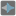• # Code is work. But don't chek test.

## Question related to missionSort Array by Element Frequency

Hi guys and hepl me please. I broke my head, phinking and trying another variants in my code. He work, but his output not check test for elements ".

```Your result:["4","4","4","4","6","6","2","2"]
Right result:[4,4,4,4,6,6,2,2]
Fail:frequency_sort([4,6,2,2,6,4,4,4])
```

I try convert my output on list, string and dict, but whenewer - don't check.

```    def frequency_sort(items):
array = {}
for i in items:
if i in array:
array[i] += 1
else:
array[i] = 1
sorted_array = sorted(array.items(), key=lambda kv: kv, reverse=True)
counter = 0
new_text = []
for i in sorted_array:
new_text.extend(str(sorted_array[counter]) * (sorted_array[counter]))
counter +=1
return new_text

if __name__ == '__main__':
print("Example:")
print(frequency_sort([4, 6, 2, 2, 6, 4, 4, 4]))

# These "asserts" are used for self-checking and not for an auto-testing
assert list(frequency_sort([4, 6, 2, 2, 6, 4, 4, 4])) == [4, 4, 4, 4, 6, 6, 2, 2]
assert list(frequency_sort(['bob', 'bob', 'carl', 'alex', 'bob'])) == ['bob', 'bob', 'bob', 'carl', 'alex']
assert list(frequency_sort([17, 99, 42])) == [17, 99, 42]
assert list(frequency_sort([])) == []
assert list(frequency_sort()) == 
print("Coding complete? Click 'Check' to earn cool rewards!")
```9